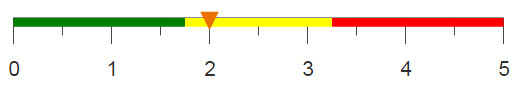# Time comparison analysis by groups

You can compare aggregated data for two different time periods, even if that data is contained in the same column.

## Difficulty## Objective

You want to perform an analysis that compares the data from one time period to a previous time period for multiple different categories. For instance, retailers often want to compare this year’s sales to last year’s sales. Additionally, you want these sales to be further broken down by group. Often, especially in time-ordered data sets, the values you want to compare are located in the same column but not in the same row. You know how to do this in SQl by using JOIN and UNION, and you would like to produce the same results using 1010data.

## SQL solution

```SELECT results.month_2015 AS `Month`,results.group_2015 AS `Group`, results.sum_sales_2015 AS `2015 Sum of Sales`,
results.sum_sales_2016 AS `2016 Sum of Sales`, results.sum_sales_2016 - results.sum_sales_2015 AS YOY,
(results.sum_sales_2016 - results.sum_sales_2015)/results.sum_sales_2015 AS `YOY Perc`
FROM (
SELECT t1.`MONTH(date)` AS month_2015 ,t1.group AS group_2015,t1.`ROUND(SUM(xsales),2)` AS sum_sales_2015,IFNULL(t2.`MONTH(date)`,
t1.`MONTH(date)`) AS month_2016,IFNULL(t2.group,t1.group) AS group_2016,IFNULL(t2.`ROUND(SUM(xsales),2)`,0) AS sum_sales_2016
FROM
(SELECT MONTH(date),YEAR(date),`group`, ROUND(SUM(xsales),2)
FROM sales_item_detail
WHERE YEAR(date)=2015
GROUP BY MONTH(date),YEAR(date),`group`)
AS t1
LEFT JOIN
(SELECT MONTH(date),YEAR(date),`group`,
ROUND(SUM(xsales),2) FROM sales_item_detail
WHERE YEAR(date)=2016
GROUP BY MONTH(date),YEAR(date),`group`)
AS t2
ON t1.group = t2.group
AND t1.`MONTH(date)` = t2.`MONTH(date)`
UNION
SELECT IFNULL(t1.`MONTH(date)`,t2.`MONTH(date)`) AS month_2014,IFNULL(t1.group,t2.group) AS group_2015,
IFNULL(t1.`ROUND(SUM(xsales),2)`,0) AS xsales_2015,t2.`MONTH(date)`,t2.group,t2.`ROUND(SUM(xsales),2)`
FROM
(SELECT MONTH(date),YEAR(date),`group`,
ROUND(SUM(xsales),2) FROM sales_item_detail
WHERE YEAR(date)=2015
GROUP BY MONTH(date),YEAR(date),`group`)
AS t1
RIGHT JOIN
(SELECT MONTH(date),YEAR(date),`group`,
ROUND(SUM(xsales),2) FROM sales_item_detail
WHERE YEAR(date)=2016
GROUP BY MONTH(date),YEAR(date),`group`)
AS t2
ON t1.group = t2.group
AND t1.`MONTH(date)` = t2.`MONTH(date)`
)
AS results
ORDER BY results.group_2015 ASC;```

Using SQL, in order to utilize results from executed select statements in additional select statements, you need to nest the statements into one query. Additionally, each of these nested select statements need to be labeled as a temporary table, using AS. With 1010data, you can execute queries consecutively, using the results of the previous query as a starting point for the next one, without having to save your results or nest your statements.

Another drawback of using SQL to complete this problem is that when you join tables together using LEFT JOIN and RIGHT JOIN, values from the joining table that do not have a match, are given a value of NULL. With 1010data, you use a <link> instead of a JOIN to combine tables in this way and values that do not match are given a value of 0. This makes it possible to still use these values in aggregations, where as aggregate functions in SQL ignore NULL values.

Note: This solution was created using MySQL where completing a FULL OUTER JOIN is not possible. Therefore, a LEFT JOIN and a RIGHT JOIN are combined with a UNION to produce the same affect.

## 1010data Macro Language solution

```<base table="pub.doc.retail.altseg.sales_detail_transid"/>
<willbe name="month" label="Month" value="month(trans_date)"/>
<willbe name="year" label="Year" value="year(trans_date)"
format="type:nocommas"/>
<tabu label="Tabulation on Sales Detail" breaks="group,month,year">
<break col="group" sort="up"/>
<break col="month" sort="up"/>
<break col="year" sort="up"/>
<tcol source="xsales" fun="sum" name="sum" label="Sum of`Extended`Sales"/>
</tabu>
<willbe name="last_year" label="Last Years Sum"
value="g_rshift(month group;;;sum;-1)" format="dec:2"/>
<willbe name="yoy" label="YOY" value="sum-last_year" format="dec:2"/>
<willbe name="yoy_perc" label="YOY Percentage" value="yoy/last_year"
format="type:pct;dec:2"/>```

Performing a time comparison analysis allows you to examine how your data changes from one time period to another. Additionally, the summarized values for each time period should be displayed in adjacent columns to allow for easy comparison between the two.

This analogue presents two methods. The most efficient way is to use the 1010data function g_rshift(G;S;O;X;N) to shift rows according to time period. This function is used to create a new column that, along with additional columns, can then be used to determine the percent loss or gain from one year to the next.

However, first two new columns representing the month and year are created to be used along with Group as break columns in a tabulation over each month/year/group combination. (Note that the sample data set, pub.doc.retail.salesdetail, only contains information for January of 2014 and 2015.) After the tabulation, the g_rshift(G;S;O;X;N) function is used to shift the rows in the worksheet so that the same month from both years in the analysis are in adjacent rows. Once the aggregations are in the same row, calculating the difference between them, and ultimately the percentage change, is simple.

## Alternate 1010data Macro Language solution

```<base table="pub.doc.retail.altseg.sales_detail_transid"/>
<willbe name="month" label="Month" value="month(date)"/>
<willbe name="fy2015" label="FY2014" value="year(date)=2015"/>
<willbe name="fy2016" label="FY2015" value="year(date)=2016"/>
<tabu label="YOY Monthly Group Sales" breaks="month,group">
<tcol source="xsales" fun="sumwd" name="sum2015" weight="fy2015"
label="2015 Sum of Monthly Group Sales"/>
<tcol source="xsales" fun="sumwd" name="sum2016" weight="fy2016"
label="2016 Sum of Monthly Group Sales"/>
</tabu>
<willbe name="yoy" label="YOY" value="sum2016-sum2015" format="dec:2"/>
<willbe name="yoy_perc" label="YOY Percentage" value="yoy/sum2015"
format="type:pct;dec:2"/>
<sort col="group" dir="up"/>```

Another solution is to compute a weighted sum in a tabulation using reference columns, one for each time period, to place the desired values in new columns in the same row. Before completing a tabulation, you must create the reference columns. One column is created to distinguish the month and two additional columns are created to distinguish which fiscal year each transaction belongs to.

A tabulation then uses the Month column along with Group to break on and group the records by. The fiscal year flag columns are then used as weights for the dot product in the calculation. Once the aggregations are in the same row, calculating the difference between them, and ultimately the percentage change, is simple.

## Further reading

If you would like to learn more about the functions and operations discussed in this recipe, click on the links below:

<tabu>